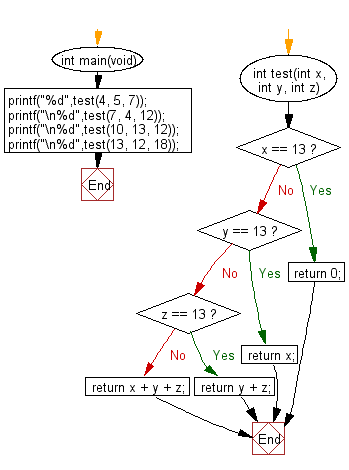﻿ C : Add the 3 integers and do not count one if it is 13# C Exercises: Compute the sum of the three integers. If one of the values is 13 then do not count it and its  right towards the sum

## C-programming basic algorithm: Exercise-30 with Solution

Write a C program to compute the sum of the three integers. If one of the values is 13 then do not count it and its right towards the sum.

C Code:

``````#include <stdio.h>
#include <stdlib.h>
int main(void){
printf("%d",test(4, 5, 7));
printf("\n%d",test(7, 4, 12));
printf("\n%d",test(10, 13, 12));
printf("\n%d",test(13, 12, 18));
}
int test(int x, int y, int z)
{
if (x == 13) return 0;
if (y == 13) return x;
if (z == 13) return y + z;
return x + y + z;
}
``````

Sample Output:

```16
23
10
0
```

Pictorial Presentation:Flowchart:C Programming Code Editor:

What is the difficulty level of this exercise?

Test your Programming skills with w3resource's quiz.

﻿

## C Programming: Tips of the Day

Why doesn't a+++++b work?

printf("%d",a+++++b); is interpreted as (a++)++ + b according to the Maximal Munch Rule!.

++ (postfix) doesn't evaluate to an lvalue but it requires its operand to be an lvalue.

! 6.4/4 says the next preprocessing token is the longest sequence of characters that could constitute a preprocessing token"

Ref : https://bit.ly/3fdldUT